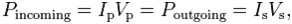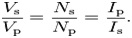# Everything You Need to Know About Transformers & Their Functioning

What is the basic component in DC or AC power supplies? Of course it is the electrical transformer. Ever wondered how do transformers work? If this question comes to your mind often, you are definitely at the right place.

But before I start, let me give a brief about transformers and different types

## What is an electrical transformer?

An electrical transformer is a static device which is used for the transformation of AC electrical signal in one circuit to the electrical signal of the same frequency in another circuit with a little loss of power. The voltage in a circuit can be increased or decreased, but with a proportional increase or decrease in the current ratings.

## Different Types of Transformers

Different types of transformers can be classified based on different criteria like function, core, etc.

Classification according to function:

Step-Up Transformer

A step up transformer is the one in which the primary voltage of the coil is lesser than secondary voltage. A Step-up transformer can be used for increasing voltage in the circuit. It is used in flexible ac transmission systems or FACTS by SVC.

Step-Down Transformer

A step-down transformer is used for reducing the voltage. The type

of transformer in which the primary voltage of the coil is greater than the secondary voltage is termed as step down transformer. Most power supplies use a step-down transformer to reduce the dangerously high voltage to a safer low voltage.

The ratio of the number of turns on each coil, called the turn’s ratio determines the ratio of the voltages. A step-down transformer has a large number of turns on its primary (input) coil which is connected to the high voltage mains supply, and a small number of turns on its secondary (output) coil to give a low output voltage.

TURNS RATIO = (Vp / Vs) = (Np / Ns) Where, Vp = primary (input) voltage Vs = secondary (output) voltage Np = number of turns on primary coil Ns = number of turns on secondary coil Ip = primary (input) current Is = secondary (output) current.

Classification according to core

1. Core type 2. Shell type

Core Type Transformer

In this type of transformer, the windings are given to the considerable part of the circuit in the core type of the transformer. The coils used are of form-wound and cylindrical type on the core type. It has a single magnetic circuit.

In core type transformer, the coils are wounded in helical layers with different layers insulated from each other by materials like mica. The core is having two rectangular limbs and the coils are placed on both the limbs in the core type.

Shell Type Transformer

Shell type transformers are the most popular and efficient type of transformers. The shell type transformer has a double magnetic circuit. The core has three limbs and both the winding are placed on the central limbs. The core encircles most parts of the winding. Generally multi-layer disc and sandwich coils are used in shell type.

Each high voltage coil is in between two low voltage coils and low voltage coils are nearest to top and bottom of the yokes. The shell type construction is mostly preferred for operating at very high voltage of transformer.

Natural cooling does not exist in the shell type transformer as the winding in the shell type is surrounded by the core itself. A large number of winding are  needed to be removed for better maintenance.

Other Types of Transformers

The types of transformers differ in the manner in which the primary and secondary coils are provided around the laminated steel core of the transformer:

• Based on winding, the transformer can be of three types

1. Two winding transformer (ordinary type) 2. Single winding (auto type) 3. Three winding (power transformer)

• Based on the arrangement of the coils the transformers are classified as:

1. Cylindrical type 2. Disc type

• According to use

1. Power transformer 2. Distribution transformer 3. Instrument transformer

Instrument transformer can subdivided into two types:

a) Current transformer b) Potential transformer

• According to the type of cooling the transformer can be of two types

1. Natural cooling 2. Oil immersed natural cooled 3. Oil immersed natural cooled with forced oil circulation

### Working of Transformer

Let us now shift our attention to our basic requirement: How do transformers work? The operation of transformer mainly works on the principle of mutual inductance between two circuits linked by a common magnetic flux. A transformer is basically used for transformation of electrical energy.

Transformers consist of types of conducting coils as primary winding and secondary windings.

The input coil is called the primary winding and the output coil is called the secondary winding of the transformer.

There is no electrical connection between the two coils; instead they are linked by an alternating magnetic field created in the soft-iron core of the transformer. The two lines in the middle of the circuit symbol represent the core. Transformers waste very little power so the power out is almost equal to the power in.

The primary coil and the secondary coil posses high mutual inductances. If one of the coils is connected to the source of alternating voltage, then an alternating flux will set up in the laminated core.

This flux gets linked up with the other coil and an electromagnetic force is induced, as per Faraday’s law of electromagnetic inductance.

e = M di/dt Where e is induced EMF M is mutual inductance

If the second coil is closed then the current in the coil is transferred from primary coil of the transformer to the secondary coil.

### Ideal power equation of transformer

While we focus on our query of how do transformers work, the basic we need to know is about the ideal power equation of transformer.

If the secondary coil is attached to a load that allows current to flow in the circuit, electrical power is transmitted from the primary circuit to the secondary circuit.

Ideally, the transformer is perfectly efficient; all the incoming energy is transformed from the primary circuit to the magnetic field and into the secondary circuit. If this condition is met, the incoming electric power must equal the outgoing power:Giving the ideal transformer equationTransformers normally have high efficiency, so this formula is a reasonable approximation.

If the voltage is increased, then the current is decreased by the same factor. The impedance in one circuit is transformed by the square of the turn’s ratio.

For example, if impedance Zs is attached across the terminals of the secondary coil, it appears to the primary circuit to have an impedance of (Np/Ns) 2 Zs. This relationship is reciprocal, so that the impedance Zp of the primary circuit appears to the secondary to be (Ns/Np) 2Zp.

We hope this article has been brief yet precisely informative about how do transformers work. Here is a simple yet important question for the readers- How is a transformer selected for designing a power supply.